Acceptance Sampling

Also found in: Dictionary, Thesaurus, Financial, Wikipedia.

acceptance sampling

[ak′sep·təns ‚sam·pliŋ]
(industrial engineering)
Taking a sample from a batch of material to inspect for determining whether the entire lot will be accepted or rejected.

Acceptance Sampling

the aggregate of the statistical methods for inspecting a mass-produced product to determine if it meets given specifications. Acceptance sampling is an effective means of ensuring the high quality of mass-produced products.

Acceptance sampling is conducted on the basis of a reference standard, or system of inspection rules. These rules prescribe the use of a certain sampling plan depending on such factors as the number of items in the lot that is being checked, the results of the inspection of earlier lots, and the laboriousness of the inspection. The chief method of selecting items for inspection is random sampling without replacement, where the items for inspection are chosen at random and any possible sample composition has an equal probability. Other methods of sampling are also occasionally used.

If the items are classified as nondefective or defective as a result of the inspection, we speak of sampling by attributes. In practice, single sampling plans by attributes are widely used in acceptance sampling. Such plans are defined by specifying the sample size—the number n of items selected for inspection—and the acceptance number c. The significance of c is that if d—the number of defective items found in the sample—is greater than c, the lot is rejected; if, however, dc, the lot is accepted. Sometimes it is advisable to use double sampling plans by attributes. The plans are defined by the sizes n1 and n2 of the first and second samples, respectively. If d1—the number of defective items found in the first sample—is not greater than c1, the lot is accepted; but if d1r1 (r1 > c1), it is rejected. When c1 < d1 < r1, a second sample containing n2 items is taken. If the total number of defective units d1 + d2 is not greater than c2, the lot is accepted; if d1 + d2 > c2, it is rejected. Multiple, sequential, or other types of sampling plans are recommended in some cases.

Under some production conditions, rejection of a lot entails inspection of all items in the lot in order to eliminate all defective articles from it; in other cases rejection means destroying the items or using the items as raw material to be recycled back into production—metal products, for example, may be melted down. With acceptance sampling the decision to accept or reject is made on the basis of an inspection of just some randomly selected items. The probability of accepting lots with defective items is, therefore, never zero. When, as in tensile tests, the inspection has a destructive character, acceptance sampling is the only possible method of acceptance control. If the properties of the articles do not change during inspection, total inspection is theoretically possible. A careful sampling inspection may produce more objective results than the necessarily less careful (owing to the larger amount of work) total inspection.

If the items are selected for inspection on the basis of random sampling, the operating characteristic of the sampling plan can be calculated. The operating characteristic equals the probability P(D) that a lot containing D defective items will be accepted. Figure 1,a shows the operating characteristics of a single sampling plan for η = 35 and c= 2; Figure 1,b of a double sampling plan for n1 = 23, n2 = 56, c1 = 0, r1 = 4, and c2 = 3; and Figure 1,c of a sequential sampling plan. For these plans, the average number of items subjected to total inspection in deciding on rejection is approximately the same when a lot of N = 1,000 items, including D = 10 defective items is checked.

The reference standards for acceptance sampling indicate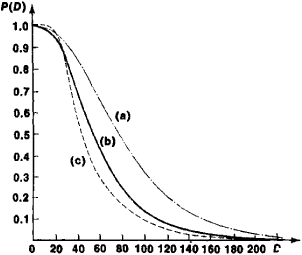Figure 1. Operating characteristics for acceptance sampling plans: (a) single sampling plan, (b) double sampling plan, (c) sequential sampling plan

what types of plans are advisable for inspecting mass-produced products. Switching from inspection with single sampling plans to more complicated plans may decrease the probability of mistakenly accepting lots that contain a large number of defective units (see Figure 1). Plans that are not of the single sampling type, however, present more difficulties with respect both to their realization and to their use as a basis for statistical estimates of the level of quality of a mass-produced product.

Suppose that D is the number of defective items in a lot and d is the number of defective items found by sampling inspection. The maximum value q of the mathematical expectation of the proportion of defective items accepted is called the average outgoing quality limit. For a single sampling plan with sample size n and acceptance number c, when items are randomly selected for inspection,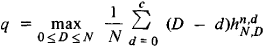Here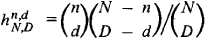is the probability of detecting d defective items in a sample of size n from a lot containing N items, and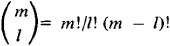If n and D are much less than N, and c is much less than n, then qρc/n, where, for example, ρ0 = 0.37, ρ1 = 85, and ρ2 = 1.40.

The cost indexes of the inspection can be used as the basis for selecting sampling plans for a series of lots. The expenses involved in acceptance sampling is the sum of the expense of inspecting the items that make up the sample and the loss from the rejection of nondefective items in the lot. The sum of expenses may also include the losses from defective items that are accepted.

The reference standards for acceptance sampling give correction rules that define the transition from normal inspection to tighter inspection and back. For example, when two of the last ten lots inspected are rejected, some reference standards recommended switching to plans with smaller values for the operating characteristic. Such a transition can be made by reducing the values of acceptance numbers or increasing sample sizes.

The inspection results can be used to obtain successive estimates for the number of defective items submitted and accepted and also for other indexes of the effectiveness of acceptance sampling. A. N. Kolmogorov has proposed methods for constructing successive estimates.

If the result of the inspection of the items is a numerical value of the measured quantity—such as size or weight—we speak of sampling by variables. Such values contain more information than do the data provided in sampling by attributes, where just the number of defective items found is given. Methods of acceptance sampling by variables can be expected to be more effective than acceptance sampling by attributes.

In the 1970’s the principles of the theory of acceptance sampling by variables were worked out on the assumption that the values being measured are mutually independent identically distributed random quantities whose distribution laws belong to a certain class—for example, the class of normal distributions. The fulfillment of these assumptions under specific conditions requires careful checking. Caution must therefore be exercised in dealing with the conclusions of the theory of acceptance sampling by variables.

Sampling by variables can be illustrated by the following example. Suppose that items are defined as nondefective if a certain dimension z is not greater than a. From the lot, we select randomly four articles, whose values for dimension z are z1, z2, z3, and z4. The lot is accepted if a —z̄ ≥ 2.5s where z̄ = (z1 + z2 + z3 + z4)/4 and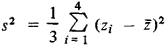Otherwise, the lot is rejected.

The rules for acceptance according to sample data have been in use for a long time. In the 19th century M. V. Ostrogradskii studied aspects of the theoretical foundations of acceptance sampling. The theory of acceptance sampling has received systematic development, however, only in the second half of the 20th century.

REFERENCES

Ostrogradskii, M. V. Poln. sobr. tr., vol 3. Kiev, 1961. Pages 215-38.
Kolmogorov, A. N. “Nesmeshchennye otsenki.” Izv. AN SSSR: Ser. malematicheskaia, 1950, vol. 14, no. 4.
Cowden, D. Statisticheskie metody kontrolia kachestva. Moscow, 1961. (Translated from English.)
Beliaev, Iu. K. Priemochnyi kontrol’ po al’ternativnomu priznaku, fase. 1-2. Moscow, 1973.
Dodge, H. F., and H. G. Romig. Sampling Inspection Tables, 2nd ed. New York-London, 1959.
Hald, A. “The Compound Hypergeometric Distribution and a System of Single Sampling Inspection Plans Based on Prior Distributions and Costs.” Technometrics, 1960, vol. 2, no. 3.

A. N. KOLMOGOROV and IU. K. BELIAEV

References in periodicals archive ?
Aslam, "Repetitive variable acceptance sampling plan for one-sided specification," Journal of Statistical Computation and Simulation, vol.
This model is also used in the acceptance sampling plans.
On the Selection of a Prior Distribution in Bayesian Acceptance Sampling.
A decisive predictive approach to the construction of sequential acceptance sampling plans for lifetimes", Applied Statistics, Vol.
They include: Measuring the Process, Statistical Tolerance Limits, Control Charts, Special-Purpose Control Charts, Product Reliability, Comparing Two Populations, Analysis of Variance, Attribute Data, Regression Analysis and Curve Fitting, Analyzing Variance Components, Experimental Design, Gage R&R Studies, Pareto Analysis, Cause-and-Effect Diagrams and Acceptance Samplings and more.

Site: Follow: Share:
Open / Close